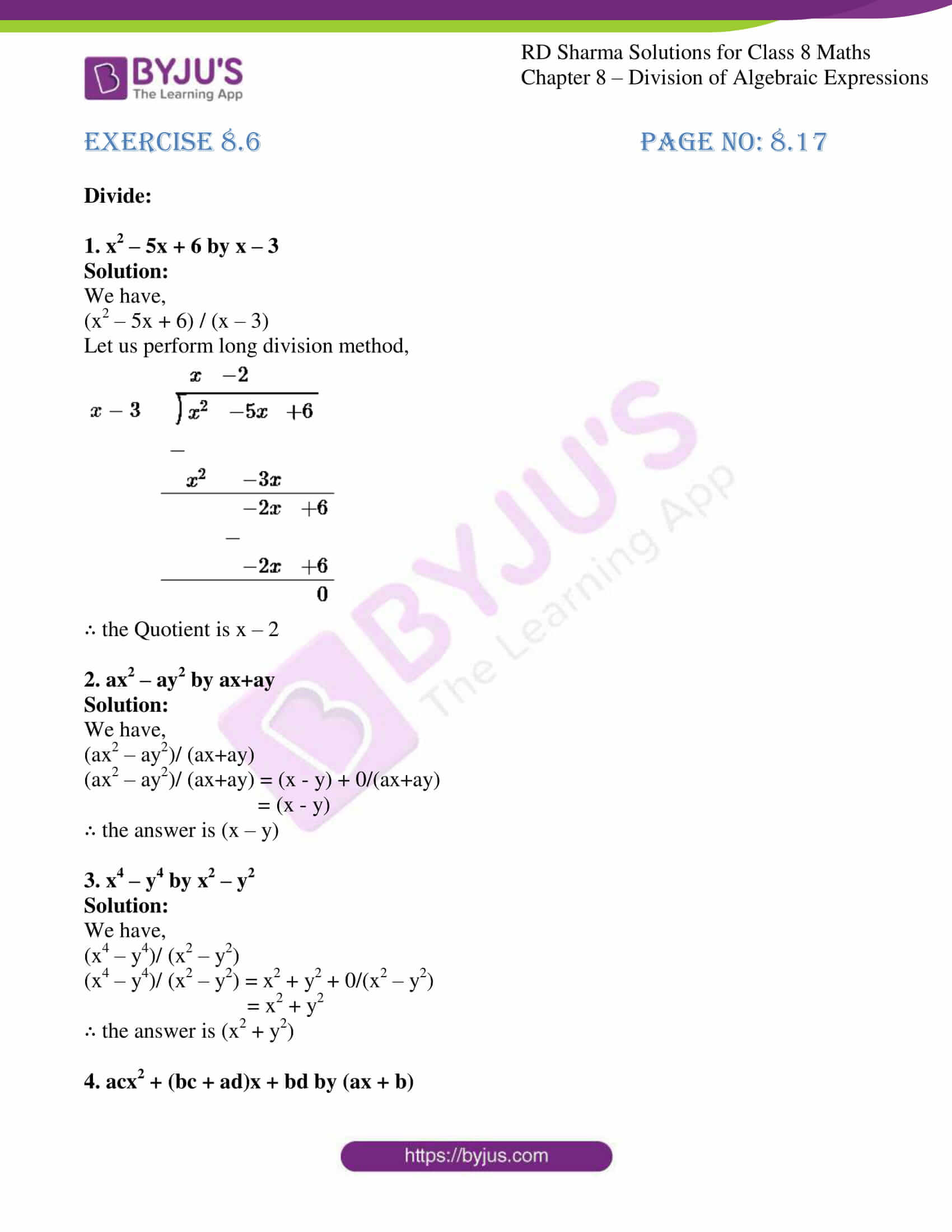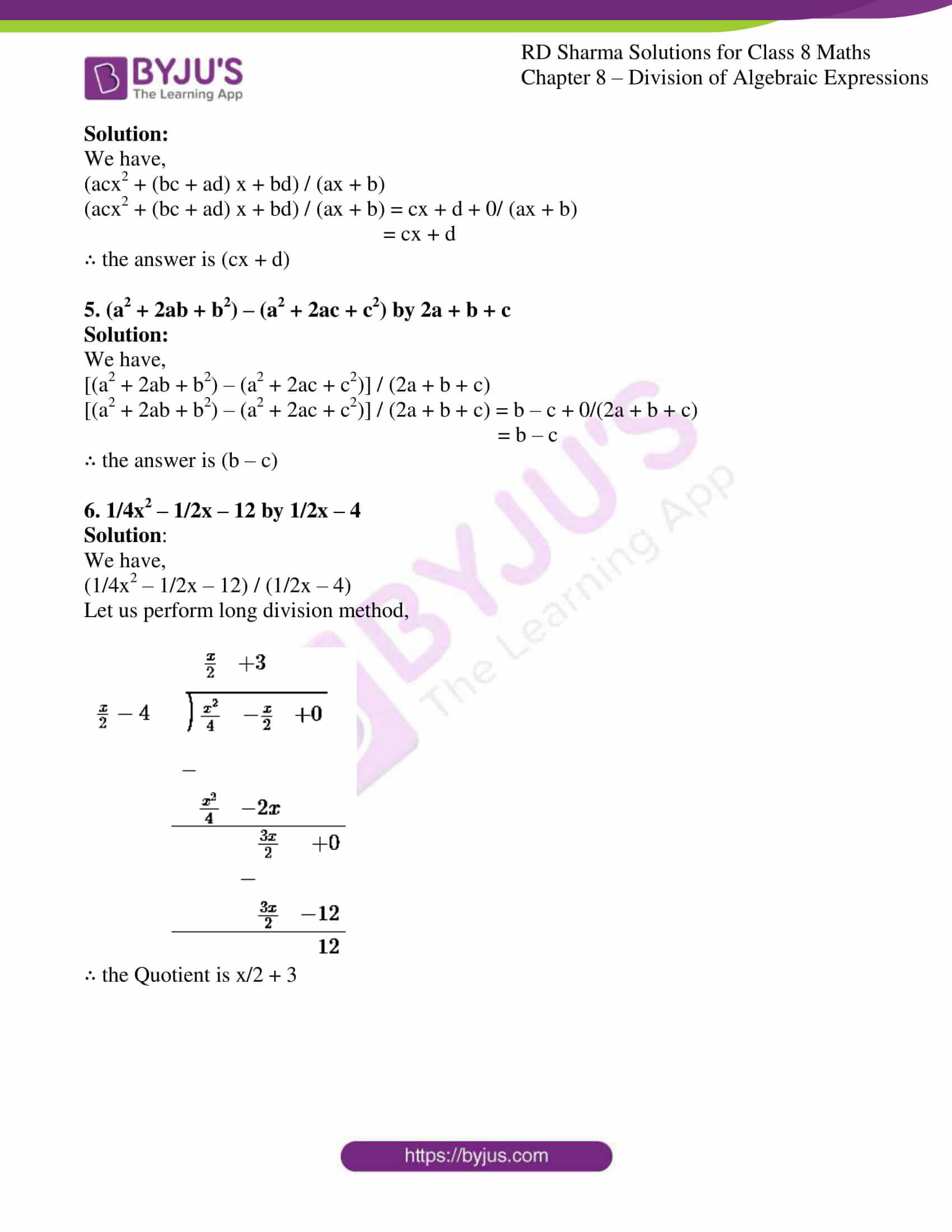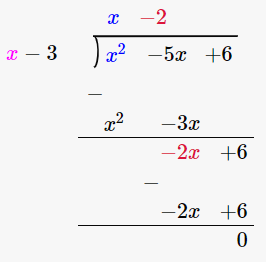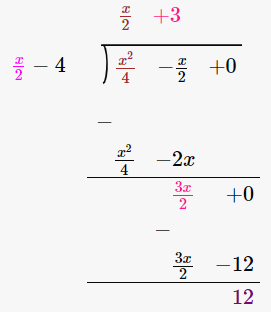# RD Sharma Solutions for Class 8 Maths Chapter 8 Division of Algebraic Expressions Exercise 8.6

We have studied factorization in the previous chapter and we shall implement it here in the form of division of polynomials by using factorization. In this Exercise 8.6 of Chapter 8, we shall learn division of polynomials in one and two variables by factorizing the dividend and divisor and then cancelling out the common factors from the numerator and denominator. Students can refer to the RD Sharma textbook to gain knowledge of the subject. To get a better understanding of the concepts students can download the RD Sharma Solutions which is prepared by our expert tutors at BYJU’S.

## Download the pdf of RD Sharma Solutions for Class 8 Maths Exercise 8.6 Chapter 8 Division of Algebraic Expressions### Access Answers to RD Sharma Solutions for Class 8 Maths Exercise 8.6 Chapter 8 Division of Algebraic Expressions

#### EXERCISE 8.6 PAGE NO: 8.17

Divide:

1. x2 – 5x + 6 by x – 3

Solution:

We have,

(x2 – 5x + 6) / (x – 3)

Let us perform long division method,∴ the Quotient is x – 2

2. ax2 – ay2 by ax+ay

Solution:

We have,

(ax2 – ay2)/ (ax+ay)

(ax2 – ay2)/ (ax+ay) = (x – y) + 0/(ax+ay)

= (x – y)

∴ the answer is (x – y)

3. x4 – y4 by x2 – y2

Solution:

We have,

(x4 – y4)/ (x2 – y2)

(x4 – y4)/ (x2 – y2) = x2 + y2 + 0/(x2 – y2)

= x2 + y2

∴ the answer is (x2 + y2)

4. acx2 + (bc + ad)x + bd by (ax + b)

Solution:

We have,

(acx2 + (bc + ad) x + bd) / (ax + b)

(acx2 + (bc + ad) x + bd) / (ax + b) = cx + d + 0/ (ax + b)

= cx + d

∴ the answer is (cx + d)

5. (a2 + 2ab + b2) – (a2 + 2ac + c2) by 2a + b + c

Solution:

We have,

[(a2 + 2ab + b2) – (a2 + 2ac + c2)] / (2a + b + c)

[(a2 + 2ab + b2) – (a2 + 2ac + c2)] / (2a + b + c) = b – c + 0/(2a + b + c)

= b – c

∴ the answer is (b – c)

6. 1/4x2 – 1/2x – 12 by 1/2x – 4

Solution:

We have,

(1/4x2 – 1/2x – 12) / (1/2x – 4)

Let us perform long division method,∴ the Quotient is x/2 + 3